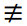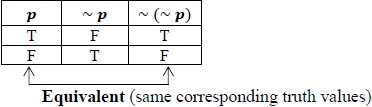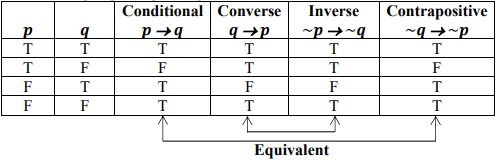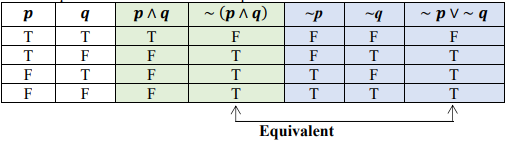# Standard #: MA.912.LT.4.5

This document was generated on CPALMS - www.cpalms.org

Determine whether two propositions are logically equivalent.

### General Information

Subject Area: Mathematics (B.E.S.T.)
Strand: Logic and Discrete Theory
Status: State Board Approved

• Equation

### Vertical Alignment

Previous Benchmarks

Next Benchmarks

### Purpose and Instructional Strategies

In Math for College Liberal Arts, students determine whether two propositions are logically equivalent using the truth values of the statements. In other courses, students will continue reinforcing the concept of equivalence and equivalent relationships.
• Students have encountered the concept of ‘equivalence’ throughout their K-12 education.
• For instance, in grade 6, students applied properties of operations to rewrite numbers in equivalent forms. In grade 7, students rewrote algebraic expressions in equivalent forms.
• Instruction includes the discussion of intuitive understanding of equivalence given concrete examples.
• For example, students are asked to discuss whether the propositional statement “It is false that the wall is not green” is logically equivalent to the statement “The wall is green” (MTR.4.1).
• In connection with MA.912.LT.4.1, students may be guided to translate the above example into symbolic statements to argue for equivalence:
~ (~p): It is false that the wall is not green.
p: The wall is green.
• Instruction includes the formal definition of equivalent statements: two propositions are logically equivalent if they always have the same truth values.
• In particular, equivalent compound statements are composed of the same simple statements and have the same corresponding truth values for any given combination of the truth values of these simple statements. In other words, if a compound statement is true, then its equivalent statement is true; if a compound statement is false, then its equivalent statement is false.
• A special symbol, ≡, is used to show that two statements are equivalent.
• For example, p ≡ ~(~p) signifies that a statement p is logically equivalent to the negation of the negated p. The symbol ? means “is equivalent to.”
• If two statements are not equivalent, this can be shown with• For example, p~p signifies that a statement p is not logically equivalent to its negation.
• In connection with MA.912.LT.4.2, instruction guides students to construct the truth tables to show that two propositional statements are equivalent.
• For example, ask students to demonstrate that p ≡ ~ (~ p) by constructing a truth table showing that the corresponding truth values are the same:• In connection with MA.912.LT.4.5, instruction reinforces the student understanding of the following logical equivalences using truth tables:
• A conditional statement and its contrapositive are logically equivalent.
• pq ≡ ~q → ~p
• The converse and inverse of a conditional statement are logically equivalent.
• qp ≡ ~p → ~q• Connecting MA.912.LT.4.1 and MA.912.LT.5.6 to instruction includes the verification and reinforcement of the equivalent relationships discussed in prior or upcoming work.
• DeMorgan’s Laws
The compound statement “~ (p AND q)” is logically equivalent to “(~p) OR (~q).”
• ~ (pq) ≡∼ p vq
The compound statement “~ (p OR q)” is logically equivalent to “(~p) AND (~q).”
• ~ (pq) ≡∼ p ∧∼ q
• Instruction guides students to verify the DeMorgan’s Laws using a truth table. For instance, students are guided to see from the truth table for ~ (pq) & ∼ p ∨ ∼ q that these statements are equivalent.### Common Misconceptions or Errors

• When the wording of a problem prompt is written as “show that statement p and statement q are equivalent,” students may incorrectly interpret “and” as a conjunction when constructing a truth table.

Instructional Task 1 (MTR.1.1, MTR.2.1, MTR.3.1
• Given two statements: pq and ∼ pq
• Part A. Show that pq is equivalent to ∼ pq
• Part B. Use the result from part A to write a statement that is equivalent to: “Do not wash that sweater or it will be ruined.”
• Part C. Given that pq ≡∼ pq, apply DeMorgan’s Law to demonstrate that ∼ (pq) ≡ p ∧ ~q. Support your work by constructing a truth table.

### Instructional Items

Instructional Item 1
• Select the statements that are equivalent to “If it is a car, then it is blue.”
• a. If it is not a car, then it is not blue.
• b. If it is not blue, then it is not a car.
• c. It is not a car or it is blue.
• d. It is a car or it is not blue. e. It is not a car and it is blue.

*The strategies, tasks and items included in the B1G-M are examples and should not be considered comprehensive.

#### Related Courses

 Course Number1111 Course Title222 7912070: Access Mathematics for Liberal Arts (Specifically in versions: 2014 - 2015, 2015 - 2018, 2018 - 2019, 2019 - 2022, 2022 - 2023, 2023 and beyond (current)) 1207350: Mathematics for College Liberal Arts (Specifically in versions: 2022 and beyond (current)) 1212300: Discrete Mathematics Honors (Specifically in versions: 2022 and beyond (current))

Printed On:9/25/2023 1:06:48 AM
Print Page | Close this window